# NCERT Solutions for Class 8 Maths Chapter 3 Understanding Quadrilaterals Ex 3.3

NCERT Solutions for Class 8 Maths Chapter 3 Understanding Quadrilaterals Exercise 3.3

Ex 3.3 Class 8 Maths Question 1.
Given a parallelogram ABCD. Complete each statement along with the definition or property used.
(ii) ∠DCB = ………
(iii) OC = ………
(iv) m∠DAB + m∠CDA = ……..Solution:
(i) AD = BC [Opposite sides of a parallelogram are equal]
(ii) ∠DCB = ∠DAB [Opposite angles of a parallelogram are equal]
(iii) OC = OA [Diagonals of a parallelogram bisect each other]
(iv) m∠DAB + m∠CDA = 180° [Adjacent angles of a parallelogram are supplementary]

Ex 3.3 Class 8 Maths Question 2.
Consider the following parallelograms. Find the values of the unknowns x, y, z.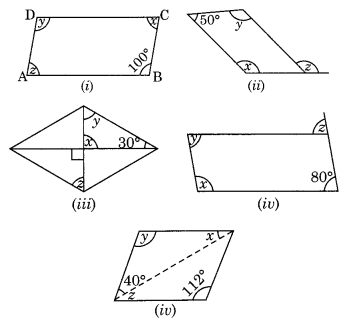Solution:
(i) ABCD is a parallelogram.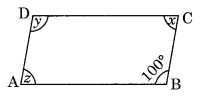∠B = ∠D [Opposite angles of a parallelogram are equal]
∠D = 100°
⇒ y = 100°
∠A + ∠B = 180° [Adjacent angles of a parallelogram are supplementary]
⇒ z + 100° = 180°
⇒ z = 180° – 100° = 80°
∠A = ∠C [Opposite angles of a ||gm]
x = 80°
Hence x = 80°, y = 100° and z = 80°
(ii) PQRS is a parallelogram.∠P + ∠S = 180° [Adjacent angles of parallelogram]
⇒ x + 50° = 180°
x = 180° – 50° = 130°
Now, ∠P = ∠R [Opposite angles are equal]
⇒ x = y
⇒ y = 130°
Also, y = z [Alternate angles]
z = 130°
Hence, x = 130°, y = 130° and z = 130°
(iii) ABCD is a rhombus.
[∵ Diagonals intersect at 90°]x = 90°
Now in ∆OCB,
x + y + 30° = 180° (Angle sum property)
⇒ 90° + y + 30° = 180°
⇒ y + 120° = 180°
⇒ y = 180° – 120° = 60°
y = z (Alternate angles)
⇒ z = 60°
Hence, x = 90°, y = 60° and z = 60°.
(iv) ABCD is a parallelogram∠A + ∠B = 180° (Adjacent angles of a parallelogram are supplementary)
⇒ x + 80° = 180°
⇒ x = 180° – 80° = 100°
Now, ∠D = ∠B [Opposite angles of a |jgm]
⇒ y = 80°
Also, z = ∠B = 80° (Alternate angles)
Hence x = 100°, y = 80° and z = 80°
(v) ABCD is a parallelogram.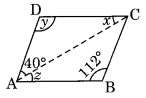∠D = ∠B [Opposite angles of a ||gm]
y = 112°
x + y + 40° = 180° [Angle sum property]
⇒ x + 112° + 40° = 180°
⇒ x + 152° = 180°
⇒ x = 180° – 152 = 28°
z = x = 28° (Alternate angles)
Hence x = 28°, y = 112°, z = 28°.

Ex 3.3 Class 8 Maths Question 3.
Can a quadrilateral ABCD be a parallelogram if
(i) ∠D + ∠B = 180°?
(ii) AB = DC = 8 cm, AD = 4 cm and BC = 4.4 cm?
(iii) ∠A = 70° and ∠C = 65°?
Solution:
(i) For ∠D + ∠B = 180, quadrilateral ABCD may be a parallelogram if following conditions are also fulfilled.
(a) The sum of measures of adjacent angles should be 180°.
(b) Opposite angles should also be of same measures. So, ABCD can be but need not be a parallelogram.
(ii) Given: AB = DC = 8 cm, AD = 4 cm, BC = 4.4 cm
In a parallelogram, opposite sides are equal.
Thus, ABCD cannot be a parallelogram.
(iii) ∠A = 70° and ∠C = 65°
Since ∠A ≠ ∠C
Opposite angles of quadrilateral are not equal.
Hence, ABCD is not a parallelogram.

Ex 3.3 Class 8 Maths Question 4.
Draw a rough figure of a quadrilateral that is not a parallelogram but has exactly two opposite angles of equal measure.
Solution:
ABCD is a rough figure of a quadrilateral in which m∠A = m∠C but it is not a parallelogram. It is a kite.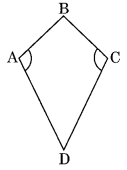Ex 3.3 Class 8 Maths Question 5.
The measures of two adjacent angles of a parallelogram are in the ratio 3 : 2. Find the measure of each of the angles of the parallelogram.
Solution:
Let ABCD is parallelogram such that
m∠B : m∠C = 3 : 2Let m∠B = 3x° and m∠C = 2x°
m∠B + m∠C = 180° (Sum of adjacent angles = 180°)
3x + 2x = 180°
⇒ 5x = 180°
⇒ x = 36°
Thus, ∠B = 3 × 36 = 108°
∠C = 2 × 36° = 72°
∠B = ∠D = 108°
and ∠A = ∠C = 72°
Hence, the measures of the angles of the parallelogram are 108°, 72°, 108° and 72°.

Ex 3.3 Class 8 Maths Question 6.
Two adjacent angles of a parallelogram have equal measure. Find the measure of each of the angles of the parallelogram.
Solution:
Let ABCD be a parallelogram in which
∠A = ∠BWe know ∠A + ∠B = 180° [Sum of adjacent angles = 180°]
∠A + ∠A = 180°
⇒ 2∠A = 180°
⇒ ∠A = 90°
Thus, ∠A = ∠C = 90° and ∠B = ∠D = 90°
[Opposite angles of a parallelogram are equal]

Ex 3.3 Class 8 Maths Question 7.
The adjacent figure HOPE is a parallelogram. Find the angle measures x, y and z. State the properties you use to find them.Solution:
∠y = 40° (Alternate angles)
∠z + 40° = 70° (Exterior angle property)
⇒ ∠z = 70° – 40° = 30°
z = ∠EPH (Alternate angle)
In ∆EPH
∠x + 40° + ∠z = 180° (Adjacent angles)
⇒ ∠x + 40° + 30° = 180°
⇒ ∠x + 70° = 180°
⇒ ∠x = 180° – 70° = 110°
Hence x = 110°, y = 40° and z = 30°.

Ex 3.3 Class 8 Maths Question 8.
The following figures GUNS and RUNS are parallelograms. Find x and y. (Lengths are in cm)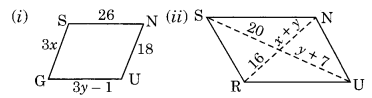Solution:
(i) GU = SN (Opposite sides of a parallelogram)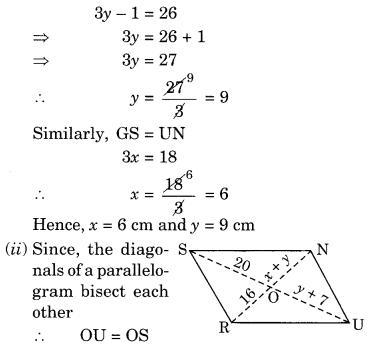⇒ y + 7 = 20
⇒ y = 20 – 7 = 13
Also, ON = OR
⇒ x + y = 16
⇒ x + 13 = 16
x = 16 – 13 = 3
Hence, x = 3 cm and y = 13 cm.

Ex 3.3 Class 8 Maths Question 9.In the above figure both RISK and CLUE are parallelograms. Find the value of x.
Solution:
Here RISK and CLUE are two parallelograms.∠1 = ∠L = 70° (Opposite angles of a parallelogram)
∠K + ∠2 = 180°
Sum of adjacent angles is 180°
120° + ∠2 = 180°
∠2 = 180° – 120° = 60°
In ∆OES,
∠x + ∠1 + ∠2 = 180° (Angle sum property)
⇒ ∠x + 70° + 60° = 180°
⇒ ∠x + 130° = 180°
⇒ ∠x = 180° – 130° = 50°
Hence x = 50°

Ex 3.3 Class 8 Maths Question 10.
Explain how this figure is a trapezium. Which of its two sides are parallel?Solution:
∠M + ∠L = 100° + 80° = 180°
∠M and ∠L are the adjacent angles, and sum of adjacent interior angles is 180°
KL is parallel to NM
Hence KLMN is a trapezium.

Ex 3.3 Class 8 Maths Question 11.
Find m∠C in below figure if $$\bar { AB }$$ || $$\bar { DC }$$
Solution:
Given that $$\bar { AB }$$ || $$\bar { DC }$$
m∠B + m∠C = 180° (Sum of adjacent angles of a parallelogram is 180°)120° + m∠C = 180°
m∠C = 180° – 120° = 60°
Hence m∠C = 60°

Ex 3.3 Class 8 Maths Question 12.
Find the measure of ∠P and ∠S if $$\bar { SP }$$ || $$\bar { RQ }$$ in figure, is there any other method to find m∠P?)Solution:
Given that ∠Q = 130° and ∠R = 90°
$$\bar { SP }$$ || $$\bar { RQ }$$ (given)
∠P + ∠Q = 180° (Adjacent angles)
⇒ ∠P + 130° = 180°
⇒ ∠P = 180° – 130° = 50°
and, ∠S + ∠R = 180° (Adjacent angles)
⇒ ∠S + 90° = 180°
⇒ ∠S = 180° – 90° = 90°
Alternate Method:
∠Q = 130°, ∠R = 90° and ∠S = 90°
We know that
∠P + ∠Q + ∠R + ∠Q = 360° (Angle sum property of quadrilateral)
⇒ ∠P + 130° + 90° + 90° = 360°
⇒ ∠P + 310° = 360°
⇒ ∠P = 360° – 310° = 50°
Hence m∠P = 50°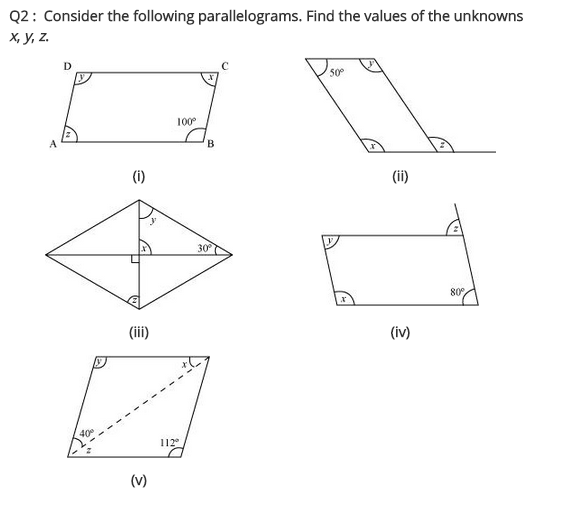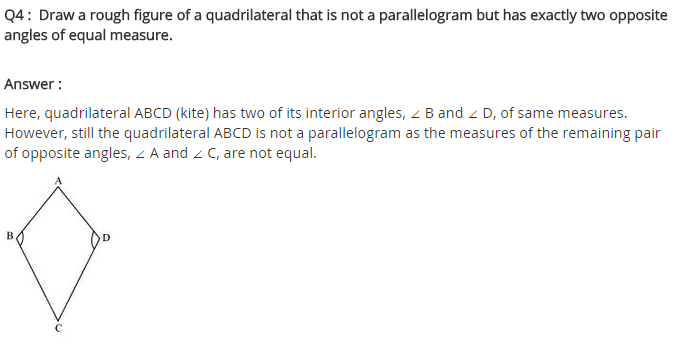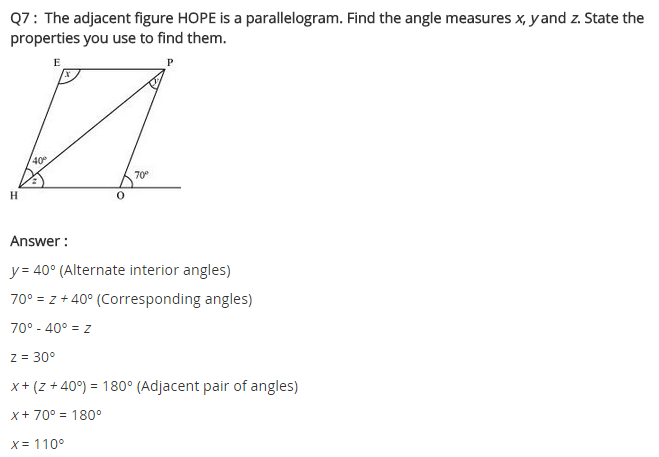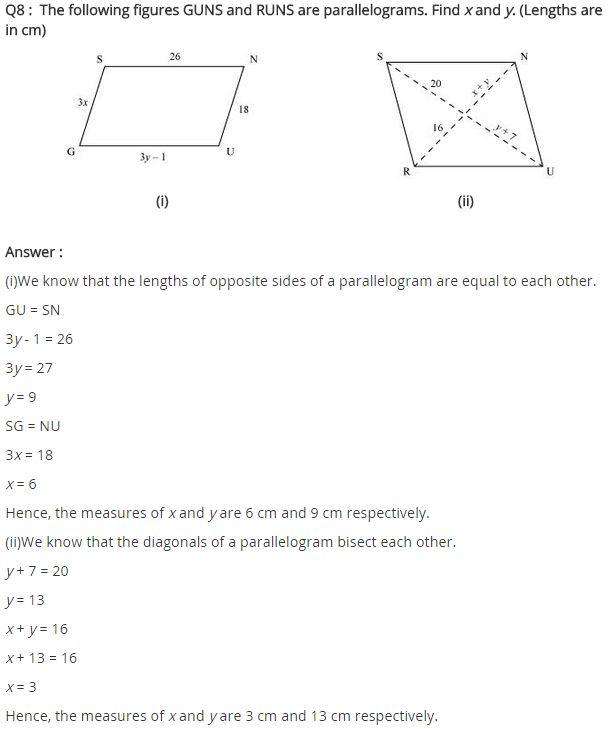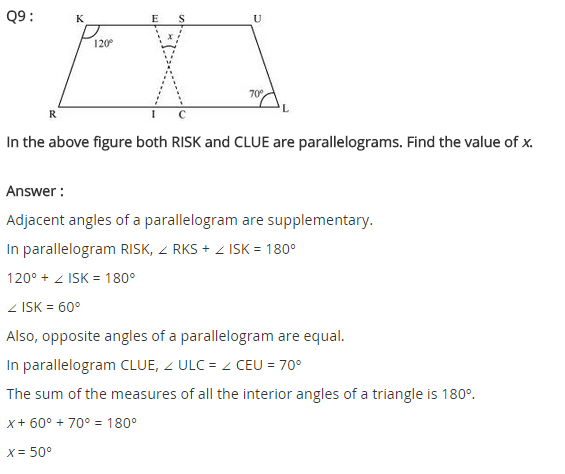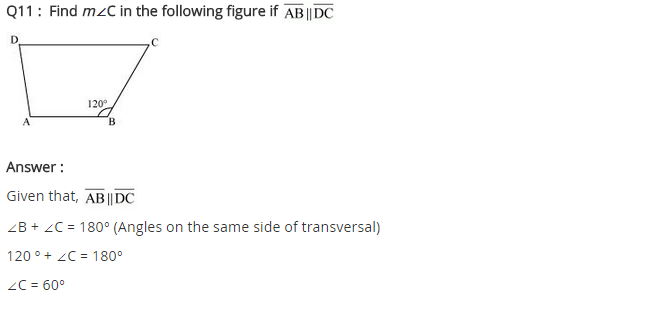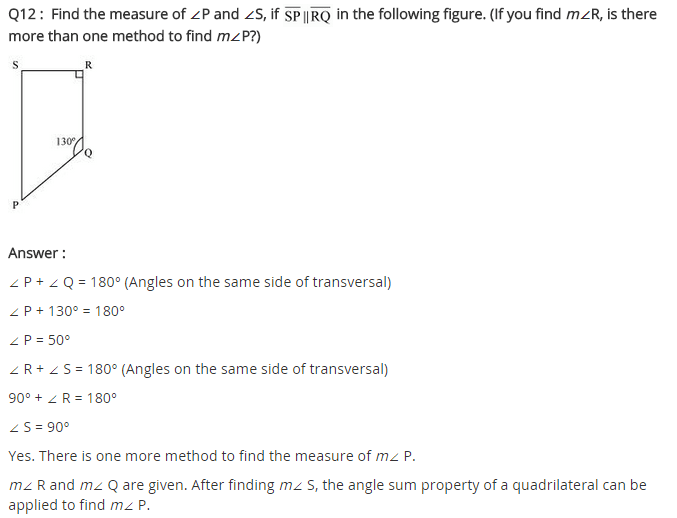+